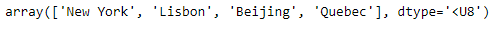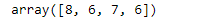Related Articles

# Find the length of each string element in the Numpy array

• Last Updated : 06 Mar, 2019

NumPy builds on (and is a successor to) the successful Numeric array object. Its goal is to create the corner-stone for a useful environment for scientific computing. NumPy provides two fundamental objects: an N-dimensional array object (ndarray) and a universal function object (ufunc).

In this post we are going to discuss ways in which we can find the length of each string element in the given numpy array.

Problem #1 : Given a numpy array whose underlying data is of string type. Find the length of each element in the given object.

Solution : We are going to use `numpy.vectorize()` function to find the length of each element in the given numpy array object.

 `# importing the numpy library as np``import` `numpy as np`` ` `# Create a numpy array``arr ``=` `np.array([``'New York'``, ``'Lisbon'``, ``'Beijing'``, ``'Quebec'``])`` ` `# Print the array``print``(arr)`

Output :Now we will use `numpy.vectorize()` function to find the length of each element in the given numpy array object.

 `# Use vectorize function of numpy``length_checker ``=` `np.vectorize(``len``)`` ` `# Find the length of each element``arr_len ``=` `length_checker(arr)`` ` `# Print the length of each element``print``(arr_len)`

Output :As we can see in the output, we have successfully calculated the length of each string element in the given numpy array object.

Problem #2 : Given a numpy array whose underlying data is of string type. Find the length of each element in the given object.

Solution : We are going to use List comprehension technique to find the length of each element in the given numpy array object.

 `# importing the numpy library as np``import` `numpy as np`` ` `# Create a numpy array``arr ``=` `np.array([``'New York'``, ``'Lisbon'``, ``'Beijing'``, ``'Quebec'``])`` ` `# Print the array``print``(arr)`

Output :Now we will use List comprehension technique to find the length of each element in the given numpy array object.

 `# Find the length of each element``arr_len ``=` `[``len``(i) ``for` `i ``in` `arr]`` ` `# Print the length of each element``print``(arr_len)`

Output :As we can see in the output, we have successfully calculated the length of each string element in the given numpy array object.

Attention geek! Strengthen your foundations with the Python Programming Foundation Course and learn the basics.

To begin with, your interview preparations Enhance your Data Structures concepts with the Python DS Course. And to begin with your Machine Learning Journey, join the Machine Learning – Basic Level Course

My Personal Notes arrow_drop_up